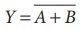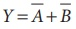Home | | Physics 12th Std | De MorganŌĆÖs Theorem

# De MorganŌĆÖs Theorem

1. De MorganŌĆÖs First Theorem, 2. De MorganŌĆÖs Second Theorem - Statement and Proof

DE MORGANŌĆÖS THEOREM

## De MorganŌĆÖs First Theorem

The first theorem states that the complement of the sum of two logical inputs is equal to the product of its complements.

### Proof

The Boolean equation for NOR gate isThe Boolean equation for a bubbled AND gate isBoth cases generate same outputs for same inputs. It can be verified using the following truth table.From the above truth table, we can conclude.

Thus De MorganŌĆÖs First Theorem is proved. It also says that a NOR gate is equal to a bubbled AND gate.

The corresponding logic circuit diagram is shown in Figure 9.47.## De MorganŌĆÖs Second Theorem

The second theorem states that the complement of the product of two inputs is equal to the sum of its complements.

### Proof

The Boolean equation for NAND gate isThe Boolean equation for bubbled OR gate isA and B are the inputs and Y is the output. The above two equations produces the same output for the same inputs. It can be verified by using the truth tableFrom the above truth table we can concludeThus De MorganŌĆÖs First Theorem is proved. It also says, a NAND gate is equal to a bubbled OR gate.

The corresponding logic circuit diagram is shown in Figure 9.48### EXAMPLE: 9 . 12

Simplify the Boolean identity

AC + ABC = AC

### Solution

Step 1: AC (1 + B) = AC.1 [OR law-2]

Step 2: AC . 1 = AC [AND law ŌĆō 2]

Therefore, AC + ABC = AC

Circuit DescriptionThus the given statement is proved.

Study Material, Lecturing Notes, Assignment, Reference, Wiki description explanation, brief detail
12th Physics : UNIT 10a : Semiconductor Electronics : De MorganŌĆÖs Theorem |